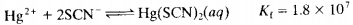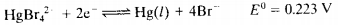### Create an Account

Home / Questions / What cathode potential versus SCE would be required to lower the total Hg II concentration...

# What cathode potential versus SCE would be required to lower the total Hg II concentration of the following solutions to 100106 M assume reaction product in each case is elemental Hg a An

What cathode potential (versus SCE) would be required to lower the total Hg (II) concentration of the following solutions to 1.00×106 M (assume reaction product in each case is elemental Hg):

(a) An aqueous solution of Hg2+?

(b) A solution with an equilibrium SCNconcentration of 0.150 M?(c) A solution with an equilibrium Brconcentration of 0.150 M?Jun 23 2020 View more View LessSubscribe To Get Solution